Numerical Investigation of Nanofluid Al2O3/Water in Elliptical Tube Equipped with Twisted Tape

Numerical Investigation of Nanofluid Al2O3/Water in Elliptical Tube Equipped with Twisted Tape

Mehrdad Hassani Mirabdolah Arash LavasaniYoon Sang Kim Mitra Ghergherehchi

Department of Mechanical Engineering, Central Tehran Branch, Islamic Azad University, Tehran 1469669191, Iran

BioComputing Laboratory, Institute for Bioengineering Application Technology, Department of Computer Science and Engineering, Korea University of Technology and Education (KOREATECH), Cheonan 31253, Republic of Korea

College of Information & Communication Engineering, School of Electronic and Electrical Engineering, Sungkyunkwan University, Suwon 16419, Republic of Korea

Corresponding Author Email:
arashlavasani@iauctb.ac.ir
Page:
520-526
|
DOI:
https://doi.org/10.18280/ijht.370219
25 October 2018
|
Accepted:
24 May 2019
|
Published:
30 June 2019
| Citation

OPEN ACCESS

Abstract:

This research was undertaken to investigate the thermal effect of Al2O3- water nanofluid flow with twisted tapes in elliptical tubes through the use of a numerical method.  Simulation was done for three-dimensional and incompressible flow with constant properties in the Reynolds range. The numerical results demonstrated that adding Al2O3 nanoparticles to water as a baseflow caused an increase in the heat transfer enhancement of the nanofluid compared to that of the base fluid. The obtained results show that simultaneous use of twisted tape with nanofluid had a better influence on heat transfer enhancement and thermal efficiency compared to the single usage of nanofluid or twisted tape, separately. Specifically, results showed that increasing the concentration of nanofluid and decreasing the twisted tape ratio caused increases in Nusselt number, thermal efficiency, and pressure.

Keywords:

elliptical tube, nanofluid, twisted tape, thermal efficiency

1. Introduction

Frequent fluids which are used for heat transfer enhancement (HTE) have a low thermal conductivity coefficient. HTE is classified into three main techniques, namely, active, passive, and compound. The active techniques require an external force such as electric field, acoustic, or surface vibration. The early studies measured suspensions of millimeter or micrometer size particles which show some enhancements, but it has problem of poor suspension stability, clogging in systems using mini and or microchannels.

An important and useful way of passively incrementing the amount of heat transfer in tubes is the use of a nanofluid instead of base fluid .

Nanoparticles increase the conductivity coefficients of these fluids due to their high conductivity and improve the amount of heat transfer in the fluid, so the use of them become very commonplace in recent years.

Another technique for passively enhancing the heat transfer in tubes is using the swirl flow devices such as Twisted Tapes (TTs) which produce secondary computation on the axial flow goes to an increase of tangential and radial turbulent vibration. [2-6]. Lee et al.  have detected the 5 % increment of Al2O3 and CuO nanofluid thermal conductivity and assess by using transient hot wire method. Choi et al achieved 160 % enhancement in thermal conductivity of 1.0 % volume concentration of CNTs in engine oil .

Nanofluid TiO2/Water which was heated in a circular horizontal tube was used to measure the heat transfer and pressure drop of turbulent flow. The results of this study showed that the heat transfer coefficient increases with increases in the volume fraction of nanoparticles and does not change with variations in Reynolds number . Heyhat et al. observed the nanofluid heat transfer coefficient increased with increases in the Reynolds number and particle concentration . Numerical analysis was carried out by Azwadi et al. to improve heat transfer in a flat tube using ethylene glycol and aluminum oxide as nanoparticles under turbulent flow conditions . Bhuiya MMK et al. have found that the Nusselt number, efficiency, and friction coefficient for a twisted tape through the pipe are higher than those for a plain tube . Simultaneous use of nanofluid and twisted tape increased heat transfer compared to single usage of nanofluid or twisted tape separately . The heat transfer was improved of a double pipe heat exchanger using aluminum/Water nanofluid and baffled twisted tape and it was observed that the rectangular baffled twisted tape performance evaluation was better than the other twisted tapes .

Another study using Fe3O4/Water nanofluid with varying cut-radius twisted tape inserts was performed in a hairpin heat exchanger. The results of this research revealed an improvement in the heat exchanger performance parameter, such as heat transfer coefficient friction factor, by increasing the volume concentration of the nanofluid .

The purpose of this research was to study the heat transfer, pressure drop, and heat efficiency in a tube with an ellipsoidal cross section under constant heat flux of 740 watts by increasing the volume fraction of Al2O3 nanoparticles and inserting twisted tapes with different twist ratios by using fluent software. It was found thermal efficiency was increased by reducing the twisted tape ratio and increasing the concentration of nanofluid.

2. Materials and Methods

2.1 Physical model

In this study, a copper tube with an elliptic cross-section was selected with a large diameter (b), a small diameter (a), and a length (L) of 27, 9, and 1000 mm, respectively. The hydraulic diameter of this pipe was Dh=24 mm and this tube was heated with constant heat flux q=740 w. Aluminum twisted tapes with twist ratios 3.125 < H/W < 18.75 were considered inside the tube. The nanofluid particles applied in this study were aluminum oxide (Al2O3, particle size changes from 1 nm to 100 nm). The physical design of the tube and the twisted tape are shown in Figures 1 (a) and 1 (b). Furthermore, the physical properties of the twist tapes are shown in Table 1.

1a.jpg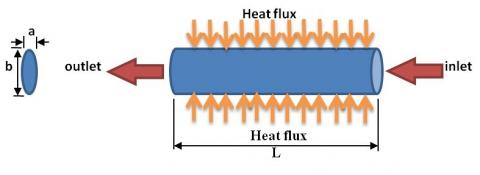(a)

1b.jpg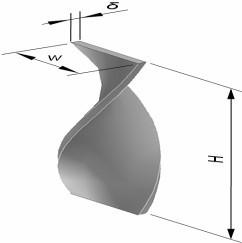(b)

Figure 1. (a) Physical image of the problem, (b) Physical view of the twisted tape inside the tube

Table 1. The physical properties of the twist tapes

 Twisted tape number W (mm) δ (mm) H (mm) Twist ratio (H/W) 1 8 1 150 18.750 2 8 1 75 9.375 3 8 1 25 3.125

W: Width, δ: Height, H: Pitch

2.2 Governing equations

The related equations of flow including mass, momentum, and energy conservation were as follows:

The mass, momentum and energy conservation equations were shown 1 to 3.

• Mass

$\operatorname{div}(p \vec{V})=0$  (1)

• Momentum

$\operatorname{div}(p \vec{V} \vec{V})=-\operatorname{gradp}+\nabla \cdot(\mu \nabla \vec{V})$  (2)

• Energy

$\operatorname{div}\left(p \vec{V} C_{p} T\right)=\operatorname{div}(K g r a d T)$  (3)

where T, P, and $\vec{V}$ were temperature, pressure, and vector of speed, respectively.

In the single-phase method for nanofluid flow, equivalent thermophysical properties for nanofluids have been introduced into the equations. The following relationships have been used in order to calculate the density, viscosity, thermal capacity, and thermal conductivity coefficient of the nanofluid.

$\rho_{n f}=\varphi \cdot \rho_{S}+(1-\varphi) \cdot \rho_{w}$  (4)

where, ρnf, ρs, and ρw are the nanofluid, nanoparticles, and water densities, respectively, and φ is the volume fraction of the nanofluid, Eq. 4.

$\mu_{n f}=\mu_{w} \cdot(1+2.5 \varphi)$               (5)

where, μnf and μw are the nanofluid and water viscosities, respectively, Eq. 5.

$C p_{n f}=\frac{\varphi .\left(\rho_{s} \cdot C p_{S}\right)+(1-\varphi) \cdot\left(\rho_{w} \cdot C p_{w}\right)}{\rho_{n f}}$     (6)

where, Cpnf, Cps, and Cpw are the specific heat capacity of nanofluids, nanoparticles, and water, respectively, Eq. 6.

$K_{n f}=\left[\frac{K_{S}+2 K_{W}+2\left(K_{S}-K_{W}\right)(1+\beta)^{3} \cdot \varphi}{K_{S}+2 K_{W}-\left(K_{S}-K_{W}\right)(1+\beta)^{3} \cdot \varphi}\right] \cdot K_{W}$      (7)

where, Knf, Ks, and Kw are the thermal conductivity of nanofluids, nanoparticles, and water, and β is the ratio of the nanolayer thickness to the average particle radius, which is considered to be 0.1, Eq. 7.

The thermophysical properties of the base fluid and the nanoparticle are in accordance with Table 2 .

Table 2. Thermophysical properties of base fluid and nanoparticle

 Substance ρ (kg/m3) Cp (J/kg.k) K (W/m.k) µ (kg/m.s) Water(w) 998.2 4182 0.6 0.001003 Al2O3(s) 3700 880 46 -

According to Table 2 and equations (4) to (7), the thermophysical properties equivalent for the nanofluid are shown in Table 3:

Table 3. Thermophysical properties for Al2O3/Water

 Nano fluid φ (%) ρ (kg/m3) Cp (J/kg.k) K (W/m.k) µ (kg/m.s) Al2O3 /Water 0.2 1003.604 4156.95 0.61770 0.00100800 Al2O3 /Water 0.5 1011.709 4120.92 0.62400 0.00101550 Al2O3 /Water 1 1025.218 4062.15 0.63600 0.00102800 Al2O3 /Water 2 1052.236 3949.13 0.66128 0.00105315

For the flowing nanofluid inside the pipe, the Reynolds number (Eq. 8), friction coefficient (Eq. 9), heat transfer coefficient (Eq.10), pressure drop (Eq. 11), and efficiency (Eq. 12) were calculated as follows [16-17]:

$R e_{n f}=\frac{\rho_{n f} \cdot D_{h} \overline{U}}{\mu_{n f}}$          (8)

where Dh is the hydraulic diameter of the pipe.

$f=\frac{64}{R e}$            (9)

$\overline{h_{n f}}=\frac{q}{A \cdot\left(T_{w}-T_{b}\right)_{M}}$         (10)

where, Tw and Tb are the wall temperature and the volume temperature of the nanofluid, respectively.

$\Delta P=\frac{f l \rho V^{2}}{2 D}$        (11)

where, f, L, and V are the friction factor, pipe length, and average velocity inside the tube, respectively.

$\eta=\left[\frac{N u_{e} / N u_{p}}{f_{e} / f_{p} )^{0.333}}\right]$     (12)

where Nue, fe, Nup, and fp are the Nusselt number and the friction coefficient, respectively, with and without the twisted tape, respectively.

2.3 Numerical solution method

The flow was considered as three-dimensional, steady, incompressible, and laminar inside the elliptical tube under constant heat flux. Continuity, momentum, and energy equations were solved after the discretization by controlling the volume and with the help of fluent software version 6.3.26 .

2.4 Production of the grid and boundary conditions

Three-dimensional grid production was done through Gambit 2.2.30 software . For a pipe with a twisted tape, a three dimensional and irregular tetrahedral mesh was used. The geometry and network of the twisted tape are as indicated in Figure 2. By considering fully-developed flow of the nanofluid, velocity inlet is applied at the input; at the output, pressure outlet and a no-slip boundary condition is applied at the solid wall (which was under constant heat flux 740 W/m2).

2.jpg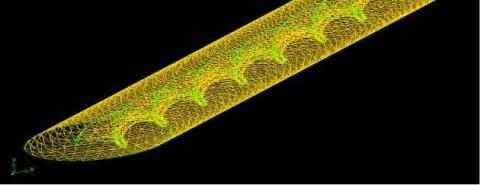Figure 2. Elliptical pipe networking with twisted tape

2.5 Mesh independency

In order to verify the independence of the number of nodes, four different types of meshes with the corresponding number of nodes (185321, 458451, 912383, and 139741) were investigated using Gambit software. For each mesh, the friction factor and Nusselt number were calculated. According to the obtained results, the number of nodes equal to 912383 was used to increase the accuracy of the calculation.

3. Results and Discussion

3.1 The validation of numerical results with experimental data

In Figure 3 (a), the effect of increasing the Reynolds number on the friction factor (in the Reynolds range 700<Re<2000) in the elliptical tube is compared with the experimental work of the Darcy–Weisbach equation. As can be seen, the results of the present study revealed an error of 0.15 to 1.8 %. Furthermore, in Figure 3 (b), the variation of the Nusselt number in terms of the Reynolds number for the elliptic tube in the Reynolds range 700<Re<2000 is compared with the experimental results of Sieder & Tate . The following comparison also showed a good agreement between the numerical and experimental results.

3a.jpg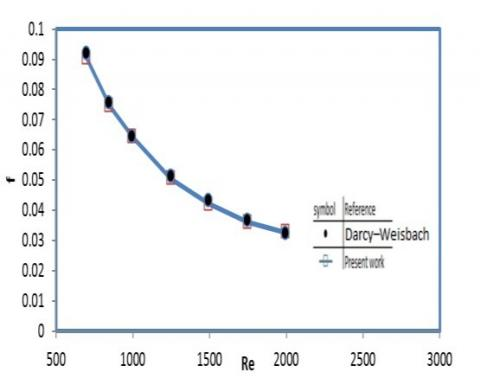(a)

3b.jpg(b)

Figure 3. (a) The effect of Reynolds number as the friction factor (a) and the Nusselt number (b)

3.2 Effect of nanofluid on pressure drop and Nusselt number

Figure 4 (a) illustrates the effect of increasing the nanofluid (Al2O3/Water) concentration on pressure drop. According to Figure 6, it can be concluded that increasing the volume fraction of nanofluid from 0.2 to 2 % causes an increase in the pressure drop from 2.1 to 5.5 %. Moreover, the effect of increasing the nanofluid (Al2O3/Water) concentration on the Nusselt number is shown in Figure 4 (b). It can be seen from this figure that by increasing the volume fraction of nanofluid from 0.2 to 2 %, the Nusselt number is increase by about 16.5 % on average.

4a.jpg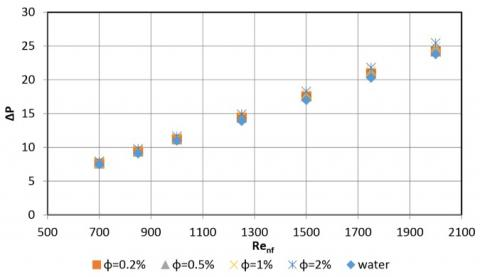(a)

4b.jpg(b)

Figure 4. Reynolds number - pressure drop (a), Reynolds number - Nusselt number (b) for different volume concentrations of nanofluid Water/Al2O3

3.3 Effect of twisted tape on pressure drop and Nusselt number

5a.jpg(a)

5b.jpg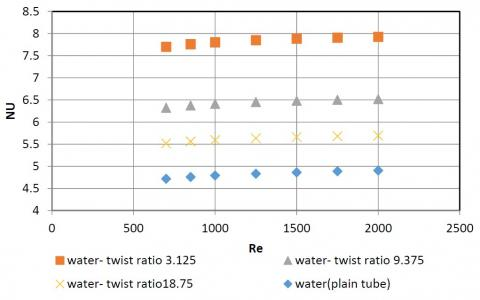(b)

Figure 5. Reynolds number - pressure drop (a), Reynolds number - Nusselt number (b) for different twisted tape ratios

Figures 5 (a) and 5 (b) illustrates the variations in pressure drop and Nusselt number with Reynolds number with different twisted tape ratios (18.75, 9.375 and 3.125). According to the numerical results, the pressure drop was increased from 192 to 223 % by reducing the twisted tape ratio from18.75 to 3.125. Furthermore, the Nusselt number was increased from 17 to 63 % by reducing the twisted ratio from 18.75 to 3.125. The effect of inserting the twisted tape in the flow path generated higher heat transfer rates than the same flow in a plain tube, resulting in a thinner thermal boundary layer along the tube wall and thus higher convective heat transfer.

3.4 Simultaneous effect of twisted tape and nanofluid on pressure drop and Nusselt number

The effects of increasing the volume concentration of nanofluid Al2O3/Water with different twisted tape ratios on pressure drop are shown in Figures 6 (a), 6 (b), and 6 (c). The results showed that reducing the twisted tape ratio from 18.75 to 3.125 and increasing the concentration of nanofluid from 0.2 to 2 % caused an increase in pressure drop from 193.8 to 238 % (compared to that of the plain tube).

6a.jpg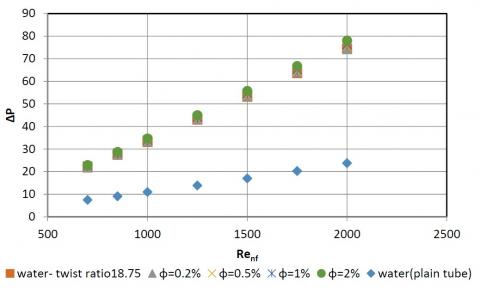(a)

6b.jpg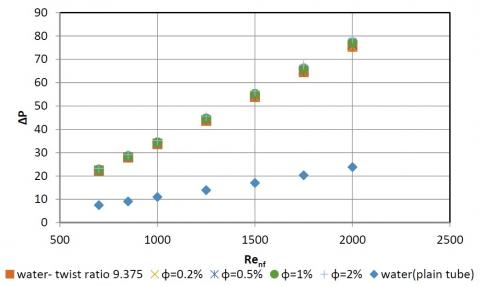(b)

6c.jpg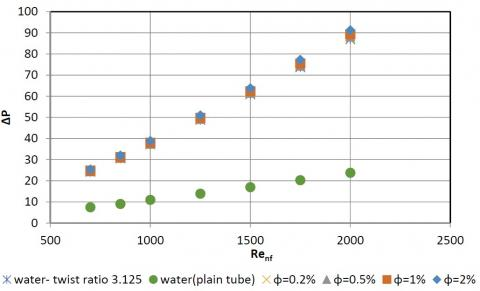(c)

Figure 6. Reynolds number - pressure drop in different twisted ratio 18.75(a), 9.375 (b) and 3.125(c) with various volume concentrations of Nanofluid Water/Al2O3

The effects of increasing the volume concentration of nanofluid Al2O3/Water with different twisted tape ratios on the Nusselt number are shown in Figures 7(a), 7(b), and 7(c). The results showed that the Nusselt number was increased from 24 to 91 % by reducing the twisted tape ratio from 18.75 to 3.125 and increasing the nanofluid concentration from 0.2 to 2 %.

7a.jpg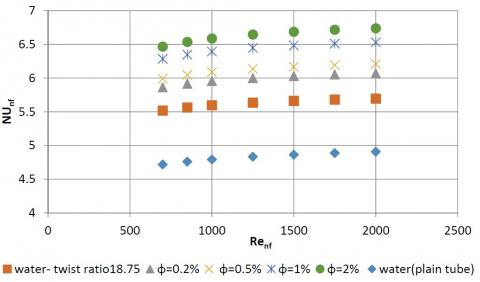(a)

7b.jpg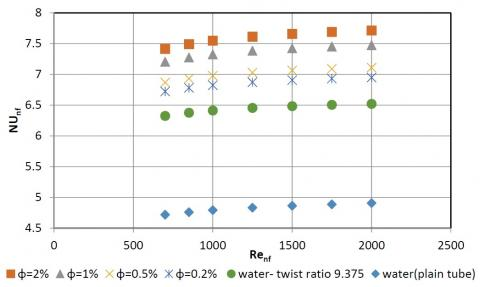(b)

7c.jpg(c)

Figure 7. Reynolds number - Nusselt number in different twisted ratio 18.75(a), 9.375 (b) and 3.125(c) with various volume concentrations of Nanofluid Water/Al2O3

3.5 Effect of nanofluid on thermal efficiency

Figure 8 illustrates the effects of increasing the nanofluid (Al2O3/Water) concentration on thermal efficiency. As can be seen, increasing the volume concentration of nanofluid from 0.2 to 2 % causes an increase in thermal efficiency from 105 to 115 %.

8.jpg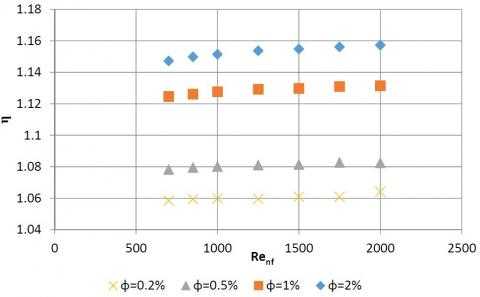Figure 8. Relation between Reynolds number and thermal efficiency for different volume concentrations of Nanofluid Water/Al2O3

3.6 Effect of twisted tape on thermal efficiency

Figure 9 shows the variations of thermal efficiency with Reynolds number in different twisted tape ratios (18.75, 9.375, and 3.125).

According to the obtained numerical results, thermal efficiency was increased from 85 to 107 % by reducing the twisted tape ratio from 18.75 to 3.125.

Furthermore, increasing the Reynolds number caused a decrease in thermal efficiency due to the insertion of twisted tape along the tube, which caused an intensive pressure drop in the flow path.

9.jpg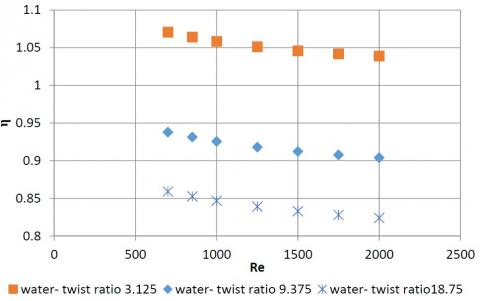Figure 9. Variations of Reynolds number and thermal efficiency for different twisted tape ratios

3.7 Simultaneous effect of twisted tape with nanofluid on thermal efficiency

The effects of increasing the volume concentration of nanofluid Al2O3/Water with different twisted tape ratios on thermal efficiency are shown in Figures 10 (a), 10 (b), and 10 (c). As is clear from the figures, thermal efficiency was increased from 91 to 123.6 % by reducing the twisted tape ratio from 18.75 to 3.125 and by increasing the concentration of nanofluid from 0.2 to 2 %.

10a.jpg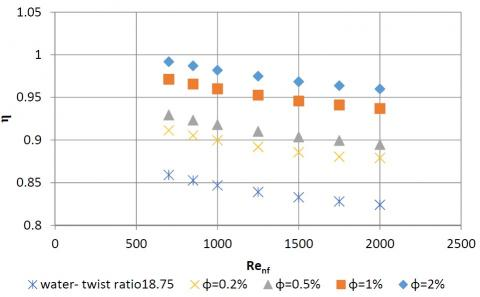(a)

10b.jpg(b)

10c.jpg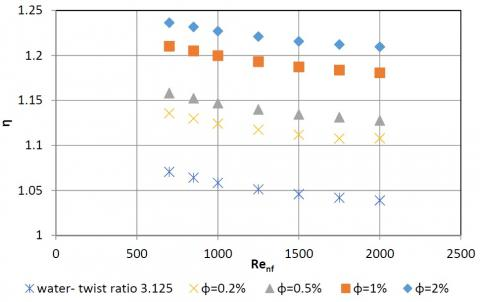(c)

Figure 10. (a) Variation of Reynolds number with thermal efficiency in diffrent twisted ratio 18.75(a), 9.375 (b) and 3.125(c) with various volume concentrations of Nanofluid Water/Al2O3

4. Conclusions

In this study, heat transfer enhancement, pressure drop, and the thermal efficiency factor of Al2O3/Water nanofluid with different volume concentrations (0<φ<2%) and different twisted tape ratios (3.125<H/W<18.75) in a laminar flow regime were numerically investigated. The highest Nusselt number was obtained in 2 % volume fraction Al2O3/Water nanofluid and a twisted tape with a twisted ratio of 3.125 and a Reynolds number of 2000. In addition, the maximum thermal efficiency was obtained around 2.1 with a Reynolds number of 700 and the simultaneous use of twisted tape with a twisted ratio of 3.125 by Al2O3/Water nanofluid in a volume fraction of 2 %. Specifically, reducing the twisted tape ratio from 18.75 to 3.125 and increasing the nanofluid concentration from 0 to 2 %, the average Nusselt number, thermal efficiency, and pressure drop were increased by around 91, 124, and 300 %, respectively. From the overall finding of this work it can be concluded that each parameter that cause enhancement in the amount of heat transfer, also increases the concentration of nanofluid make more pressure drop in tubes.

Acknowledgment

This work has supported by the National Research Foundation of Korea (NRF) grant funded by the Korea government (MSIT) (No. NRF-2017R1A2B2011989)

References

 Choi, S.U.S. (1995). Enhancing thermal conductivity of fluid with nanoparticles. In: Siginer, D.A., Wang, H.P. (Eds.), Developments and Applications of Non-Newtonian Flows, FED-V.231/ MD-V.66. ASME, New York, 99–105.

 Saha, S.K., Dutta, A., Dhal, S.K. (2001). Friction and heat transfer characteristics of laminar swirl flow through a circular tube fitted with regularly spaced twisted tape elements. International Journal of Heat and Mass Transfer, 44(22): 4211–4223. https://doi.org/10.1016/S0017-9310(01)00077-1

 Ray, S., Date, A.W. (2003). Friction and heat transfer characteristics of flow through square duct with twisted tape insert. International Journal of Heat and Mass Transfer, 46(5): 889–902. https://doi.org/10.1016/S0017-9310(02)00355-1

 Mulla, A.M., Jangamashetti, U., Manoj, Kiran. (2014). Experimental study on heat transfer and friction factor characteristics in laminar flow through tubes of shell and tube heat exchanger fitted with twisted tapes with baffles. International Journal of Research in Engineering and Technology, 3(5): 426–431.

 Sayam Sundar, L., Sharma, K.V. (2010). Turbulent heat transfer and friction factor of Al2O3 Nanofluid in circular tube with twisted tape inserts. International Journal of Heat and Mass Transfer, 53(7-8): 1409–1416. https://doi.org/10.1016/j.ijheatmasstransfer.2009.12.016

 Safikhani, H., Abbasi, F. (2015). Numerical study of nanofluid flow in flat tubes fitted with multiple twisted tapes. Advanced Powder Technology, 26(6): 1609–1617. https://doi.org/10.1016/j.apt.2015.09.002

 Lee, S., Choi, S.U.S., Li, S., Eastman, J.A. (1999). Measuring thermal conductivity of fluids containing oxide nanoparticles. Journal of Heat Transfer, 121(2): 280–289. https://doi.org/10.1115/1.2825978

 Choi, S.U.S., Zhang, Z.G., Yu, W., Lockwood, F.E., Grulke, E.A. (2001). Anomalous thermal conductivity enhancement in nanotube suspensions. Applied Physics Letters, 79(14): 2252–2254. https://doi.org/10.1063/1.1408272

 Kayhani, M.H., Soltanzadeh, H., Heyhat, M.M., Nazari, M., Kowsary, F. (2012). Experimental study of convective heat transfer and pressure drop of TiO2/water nanofluid. International Communications in Heat and Mass Transfer, 39(3): 456-462. https://doi.org/10.1016/j.icheatmasstransfer.2012.01.004

 Heyhat, M.M., Kowsary, F., Rashidi, A.M., Momenpour, M.H., Amrollahi, A. (2013). Experimental investigation of laminar convective heat transfer and pressure drop of water-based Al2O3 nanofluids in fully developed flow regime. Experimental Thermal and Fluid Science, 44: 483-489. https://doi.org/10.1016/j.expthermflusci.2012.08.009

 Azwadi, N., Sidik, C., Witri, M.N.A., Yazid, M., Azmi, W.H., Hind, M.K. (2016). Experimental and numerical investigation of heat transfer augmentation using Al2O3-ethylene glycol nanofluids under turbulent flows in a flat tube. Jurnal Teknologi (Sciences and Engineering), 78: 39-44. https://doi.org/10.1051/matecconf/201822501019

 Bhuiya, M.M.K., Ahamed, J.U., Chowdhury, M.S.U., Sarkar, M.A.R., Salam, B., Saidur, R., Kalam, M.A. (2012). Heat transfer enhancement and development of correlation for turbulent flow through a tube with triple helical tape inserts. International Communications in Heat and Mass Transfer, 39(1): 94-101. https://doi.org/10.1016/j.icheatmasstransfer.2011.09.007

 Wongcharee, K., Eiamsa-Ard, S. (2011). Enhancement of heat transfer using CuO/water nanofluid and twisted tape with alternate axis. International Communications in Heat and Mass Transfer, 38(6): 742-748. https://doi.org/10.1016/j.icheatmasstransfer.2011.03.011

 Sreekumar, P.C., Krishnamoorthy, K., Ratheesh, R. (2015). Experimental analysis on heat transfer enhancement of double pipe heat exchanger using alumina/water nanofluid and baffled twisted tape inserts. Applied Mechanics & Materials, 752-753: 458-465. https://doi.org/10.4028/www.scientific.net/AMM.752-753.458

 Krishna Varma, K.P.V., Kishore, P.S., Durga Prasad, P.V. (2017). Enhancement of heat transfer using Fe3O4/water nanofluid with varying cut-radius twisted tape inserts. International Journal of Applied Engineering Research, 12(18): 7088-7095.

 Heris, S.Z., Nassan, T.H., Noie, S.H., Sardarabadi, H., Sardarabadi, M. (2013). Laminar convective heat transfer of Al2O3/water nanofluid through square cross-sectional duct. International Journal of Heat and Fluid Flow, 44: 375-382. https://doi.org/10.1016/j.ijheatfluidflow.2013.07.006

 Kapse, A.A., Dongarwar, P.R., Gawande, R.R. (2014). Effect of helical screw tape insertion on heat transfer and pressure drop characteristics in a horizontal concentric double tube heat exchanger. Applied Mechanics and Materials, 541: 622-627. https://doi.org/10.4028/www.scientific.net/AMM.541-542.622

 FLUENT 6.3 User's Guide, https://www.sharcnet.ca/Software/Fluent6/html/ug/main_pre.htm, accessed on 10 June 2019.

 GAMBIT 2.2 User’s Guide, https://www.ansys.com/Products/Fluids/ANSYS-Fluent, accessed on 10 June 2019.

 Sieder, E.N., Tate, G.E. (1936). Heat transfer and pressure drop of liquids in tubes. Industrial & Engineering Chemistry Research, 28(12): 1429-1435. https://doi.org/10.1021/ie50324a027